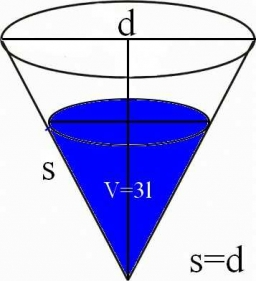# Cone-shaped 44161

How many square meters of roofing is needed to cover the cone-shaped roof, if the perimeter of its base is 15.7m and a height of 30dm

S =  30.6489 m2

### Step-by-step explanation:Did you find an error or inaccuracy? Feel free to write us. Thank you!

Tips for related online calculators
The Pythagorean theorem is the base for the right triangle calculator.

#### You need to know the following knowledge to solve this word math problem:

We encourage you to watch this tutorial video on this math problem: